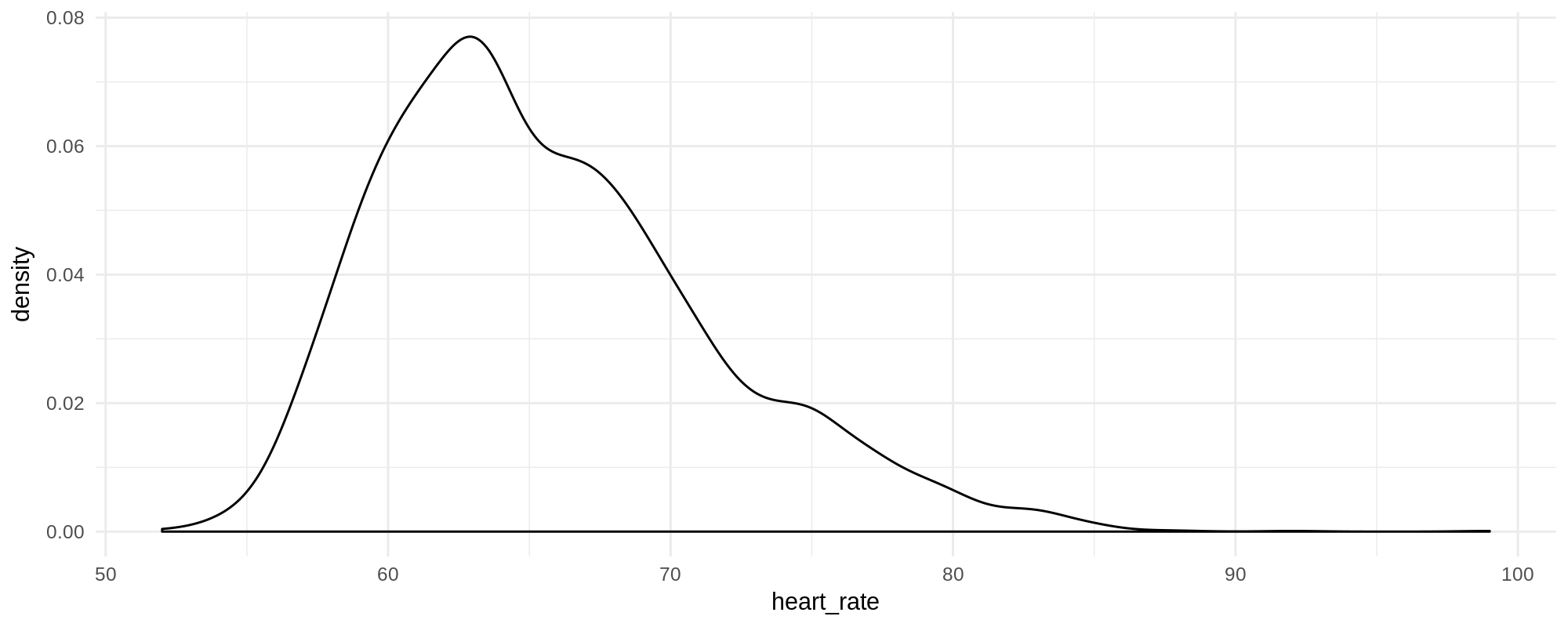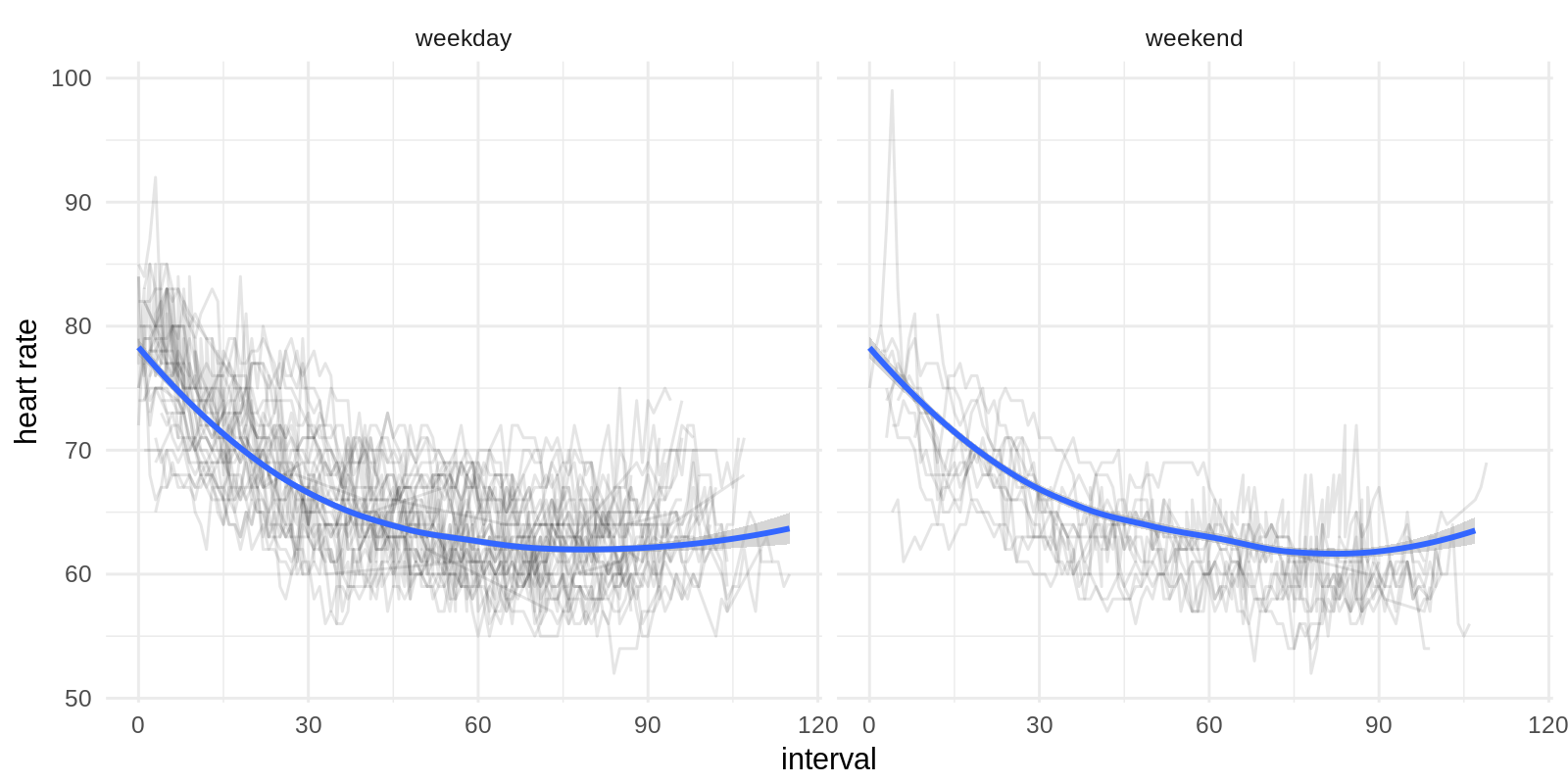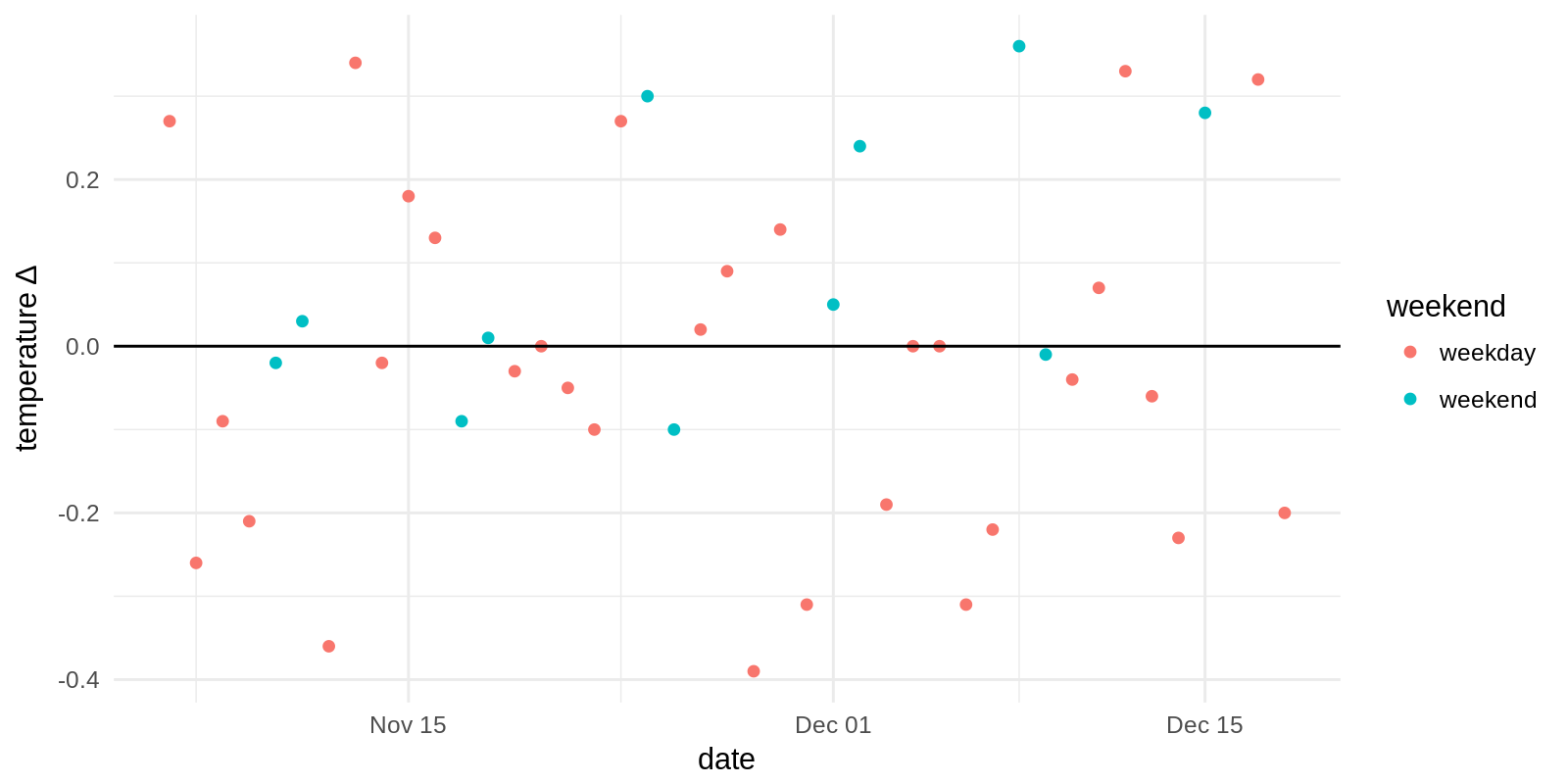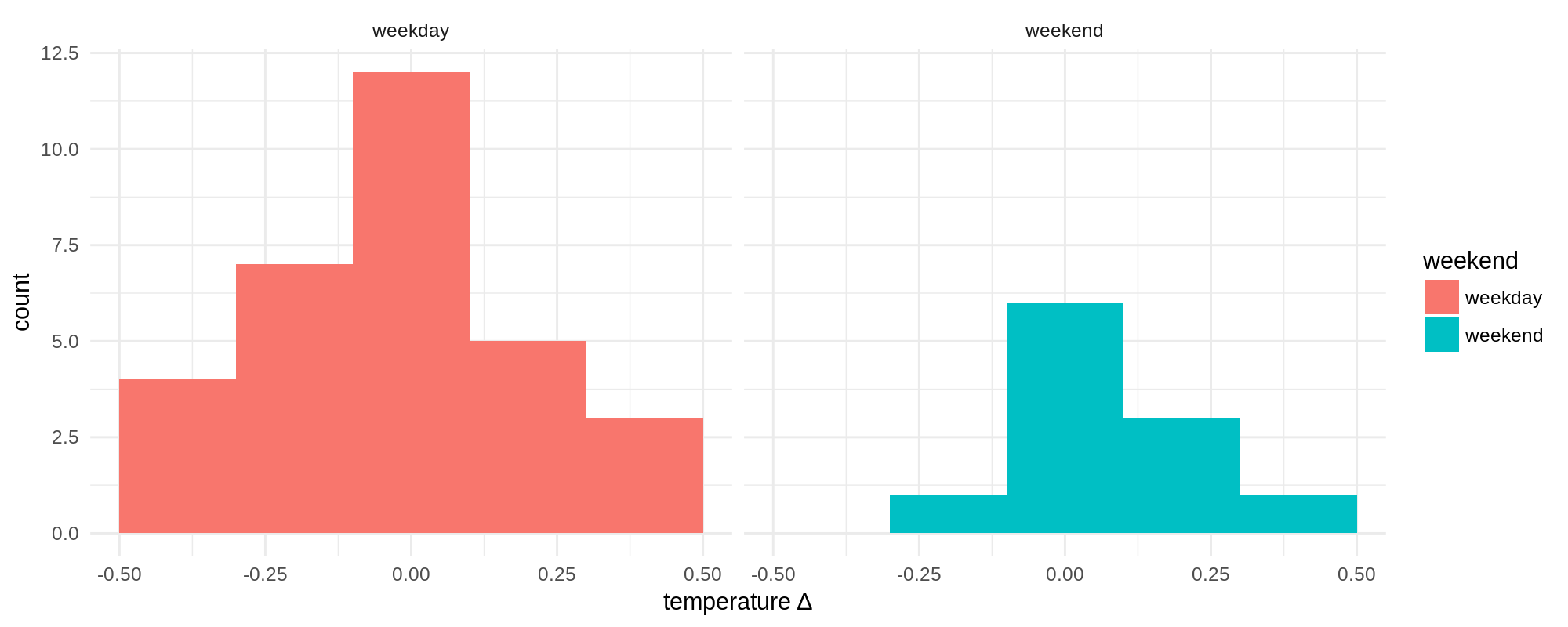# Details for `oura-body-temperature-heart-rate.ipynb`

#### Description

A notebook that analyses your Heart Rate and Body temperature data from your Oura Ring

#### Tags & Data Sources

body temperature heart rate activity sleep Oura Connect

These are useful programs. Is there anymore insights you've generated and share more python programs? Thanks!

# Looking into the Data collected by your Oura Ring¶

The Oura Ring allows you to measure vital signs during sleep such as heart rate and temperature. Here we will look into how we can visualize this data.

As usual, below comes the boilerplate to find the actual Oura data on Open Humans. Don't worry much about this at this point. If you haven't connected your Oura account to Open Humans this will fail. Go here to connect your account.

In :
```from ohapi import api
import os
import requests
import tempfile

user_details = api.exchange_oauth2_member(os.environ.get('OH_ACCESS_TOKEN'))
for i in user_details['data']:
if i['source'] == 'direct-sharing-184' and i['basename'] == 'oura-data.json':
break
import json
```
```{'id': 613774, 'basename': 'oura-data.json', 'created': '2018-12-20T00:01:23.868874Z', 'download_url': 'https://open-humans-production.s3.amazonaws.com/member-files/direct-sharing-184/638807d4-03ea-11e9-b9ba-9ae77634d62c/oura-data.json?AWSAccessKeyId=AKIAIKNTFUJJTNS6N7HA&Signature=piLYIgp7aV%2FsoppRPI7EqlfZ07U%3D&Expires=1545333134', 'metadata': {'tags': ['oura', 'activity', 'temperature', 'sleep'], 'description': 'Oura records'}, 'source': 'direct-sharing-184'}
```

Now that we have stored our data in the `oura` variable, what's our Oura profile and what are the data we have?

In :
```print('we have data on the following things:')
print(oura.keys())
print('\nour profile is:')
print(oura['profile'])
```
```we have data on the following things:

our profile is:
{'height': 180, 'weight': 65, 'gender': 'male', 'age': 33, 'user_id': 'FGNPISER7IN7W4N3KTYVVFAI5EONLDXB', 'date': '2018-12-19'}
```

Besides our `profile` we can ask for our `sleep`, our `activity` and our `readiness`. Now comes the obligatory data conversion to make this JSON file into a nice table. We don't use much of it for now, but rather stick to the 5 minute heart-rate intervals:

In :
```dates = []
hrs = []
interval = []
for sdate in oura['sleep']:
if 'hr_5min' in sdate.keys():
for i,hr_val in enumerate(sdate['hr_5min']):
interval.append(i)
hrs.append(hr_val)
dates.append(sdate['summary_date'])
import pandas as pd
dataframe = pd.DataFrame(
data = {
'date': dates,
'heart_rate': hrs,
'interval': interval
}
)
```

Post-conversion our table looks like this

In :
```dataframe.tail()
```
Out:
date heart_rate interval
4132 2018-12-18 0 86
4133 2018-12-18 64 87
4134 2018-12-18 67 88
4135 2018-12-18 0 89
4136 2018-12-18 0 90

## Heart Rate intervals measured during sleep¶

Let's check the nightly heart rate that's measured in 5 minute intervals. What's the distribution of it?

In :
```%load_ext rpy2.ipython
```
In :
```%%R -i dataframe -w 10 -h 4 --units in -r 200
library(ggplot2)

ggplot(subset(dataframe,dataframe\$heart_rate>0), aes(heart_rate)) + geom_density() + theme_minimal()
```Looks somewhat poisson-ic to me, with a peak at around 62-63 beats per minute. But let's see how these values differ over time 5 minutes interval starting off with going to sleep into later at the night and whether there's some difference between weekdays and weekends:

(DEPENDING ON HOW MUCH DATA YOU HAVE IT MIGHT TAKE A WHILE TO PRODUCE THE PLOT BELOW!)

In :
```%%R -i dataframe -w 8 -h 4 --units in -r 200
library(ggplot2)
library(lubridate)
library(plyr)
dataframe <- subset(dataframe,dataframe\$heart_rate > 0 )
dataframe\$date <- as.Date(dataframe\$date)
dataframe\$weekday <- wday(dataframe\$date, label=TRUE)
dataframe\$year <- year(dataframe\$date)
dataframe\$weekend <- dataframe\$weekday %in% c('Sun','Sat')

dataframe\$weekend <- ifelse(dataframe\$weekend == TRUE, "weekend", "weekday")
ggplot(dataframe,aes(dataframe\$interval, dataframe\$heart_rate,group=date)) +
geom_line(alpha=0.1) +
stat_smooth(data=dataframe,aes(interval,heart_rate,group=weekend), method='loess') +
theme_minimal() + facet_grid(. ~ weekend) + scale_x_continuous('interval') + scale_y_continuous('heart rate')
```We see, there's a lot of noise over all the nights we tracked our heart rate during sleep, but there seems to be a minor trend for the Heart Rate to drop in later measured intervals, just as Oura themselves highlight.

# Body Temperature¶

Another kind of data the Oura Ring is collecting is your Body Temperature, giving you one relative measurement per day. E.g. did your Body Temperature today increase or decrease in comparison to the historic data?

To look into those we can first extract those data from the `oura` variable that contains all of our data:

In :
```sleep_dates = []
temperature_delta = []
for i in oura['sleep']:
sleep_dates.append(i['summary_date'])
temperature_delta.append(i['temperature_delta'])

sleep_df = pd.DataFrame(
data = {
'date': sleep_dates,
'temperature_delta': temperature_delta,
}
)
```

With this we can now plot our body temperature variation over time and see whether we find any trends. To also look in Weekend/Weekday variability we also annotate the dates again:

In :
```%%R -i sleep_df -w 8 -h 4 --units in -r 200
library(ggplot2)
library(lubridate)
library(plyr)

sleep_df\$date <- as.Date(sleep_df\$date)
sleep_df\$weekday <- wday(sleep_df\$date, label=TRUE)
sleep_df\$year <- year(sleep_df\$date)
sleep_df\$weekend <- sleep_df\$weekday %in% c('Sun','Sat')

sleep_df\$weekend <- ifelse(sleep_df\$weekend == TRUE, "weekend", "weekday")

ggplot(sleep_df,aes(sleep_df\$date, sleep_df\$temperature_delta,color=weekend)) +
geom_point(alpha=1) + theme_minimal() + geom_hline(yintercept=0) + scale_x_date('date') + scale_y_continuous('temperature ∆')
```That looks pretty much all over the place at first glance, but it looks like there are less pronounced body temperature drops on the weekend somehow? Let's look at a distribution of the values for both conditions.

In :
```%%R -w 10 -h 4 --units in -r 200

ggplot(sleep_df, aes(temperature_delta, fill=weekend)) +
geom_histogram(binwidth=0.2) +
theme_minimal() + facet_grid(. ~ weekend) + scale_x_continuous('temperature ∆')
```With so little data it's hard to say whether this a real difference or just a matter of the much smaller sample size for weekends. So I guess more data will be needed before being able to make a judgement here!

In [ ]:
```
```